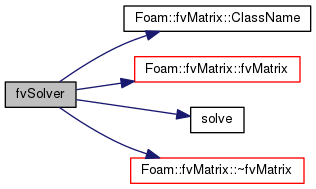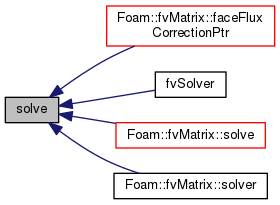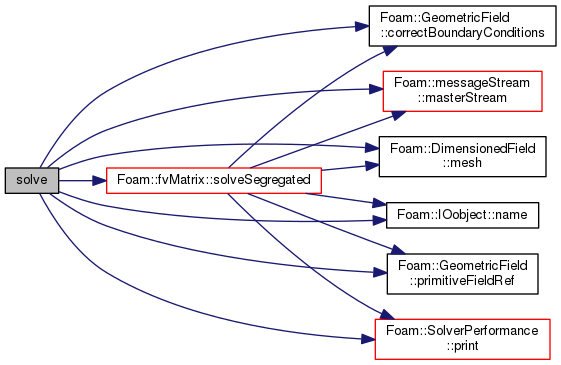The OpenFOAM Foundation
fvMatrix< Type >::fvSolver Class Reference

Solver class returned by the solver function. More...

## Public Member Functions

fvSolver (fvMatrix< Type > &fvMat, autoPtr< lduMatrix::solver > sol)

SolverPerformance< Type > solve (const dictionary &)
Solve returning the solution statistics. More...

SolverPerformance< Type > solve ()
Solve returning the solution statistics. More...

template<>
Foam::solverPerformance solve (const dictionary &solverControls)

template<>
solverPerformance solve (const dictionary &)

## Detailed Description

### template<class Type> class Foam::fvMatrix< Type >::fvSolver

Solver class returned by the solver function.

used for systems in which it is useful to cache the solver for reuse e.g. if the solver is potentially expensive to construct (AMG) and can be used several times (PISO)

Definition at line 220 of file fvMatrix.H.

## Constructor & Destructor Documentation

 fvSolver ( fvMatrix< Type > & fvMat, autoPtr< lduMatrix::solver > sol )
inline

Definition at line 230 of file fvMatrix.H.

Here is the call graph for this function:## Member Function Documentation

 SolverPerformance solve ( const dictionary & )

Solve returning the solution statistics.

Use the given solver controls

 Foam::SolverPerformance< Type > solve ( )

Solve returning the solution statistics.

Solver controls read from fvSolution

Definition at line 296 of file fvMatrixSolve.C.

Here is the caller graph for this function:Foam::solverPerformance solve ( const dictionary & solverControls )

Definition at line 98 of file fvScalarMatrix.C.

Here is the call graph for this function:solverPerformance solve ( const dictionary & )

The documentation for this class was generated from the following files: